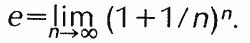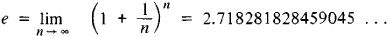# E

(redirected from e-)
Also found in: Dictionary, Thesaurus, Medical, Financial, Acronyms, Wikipedia.

## E,

fifth letter of the alphabetalphabet
[Gr. alpha-beta, like Eng. ABC], system of writing, theoretically having a one-for-one relation between character (or letter) and phoneme (see phonetics). Few alphabets have achieved the ideal exactness.
. It is a usual symbol for a mid-front vowel, such as ĕ in the English step. A mid-front vowel was represented by Greek epsilon [Gr.,=e without the aspirate], to which E corresponds in form and place (see also HH,
8th letter of the alphabet. It is a usual symbol for a glottal spirant, murmured (as in the English house) or voiceless (as in the English herb). In some Greek alphabets eta, the long e, had this form. In chemistry H is the symbol for the element hydrogen.
). English ē is pronounced as a diphthong of ĭ and y. In musical notationmusical notation,
symbols used to make a written record of musical sounds.

Two different systems of letters were used to write down the instrumental and the vocal music of ancient Greece. In his five textbooks on music theory Boethius (c.A.D. 470–A.D.
E represents a note in the scale.

## e,

in mathematics, irrational numbernumber,
entity describing the magnitude or position of a mathematical object or extensions of these concepts. The Natural Numbers

Cardinal numbers describe the size of a collection of objects; two such collections have the same (cardinal) number of objects if their
occurring widely in mathematics and science, approximately equal to the value 2.71828; it is the base of natural, or Naperian, logarithmslogarithm
[Gr.,=relation number], number associated with a positive number, being the power to which a third number, called the base, must be raised in order to obtain the given positive number.
. The number e is defined as the limit of the expression (1+1/n)n as n becomes infinitely large, orIn 1873 the French mathematician C. Hermite proved that e was transcendental, i.e., not a rootroot,
in mathematics, number or quantity r for which an equation f(r)=0 holds true, where f is some function. If f is a polynomial, r is called a root of f; for example, r=3 and r
of any algebraic equation; this proof constituted a great contribution to the growth of mathematics. The number e is also known as Euler's number, for Leonhard Euler, who discovered the famous formula eiπ=−1, where i=√−1, thus expressing the relationship between the numbers e, i, and π. The exponential function ex, often written exp(x), occurs in various applications ranging from statistics to nuclear physics.

### Bibliography

See study by E. Maor (1994).

## E

(in Russian, Napier number), the limit of the expression [1 + (1/n)]n as n increases without bound:It is the base of the natural system of logarithms. The number e is a transcendental number; this fact was first proved in 1873 by C. Hermite. Naming the number e after J. Napier is not entirely valid. (See.)

## e

(mathematics)
The base of the natural logarithms; the number defined by the equation approximately equal to 2.71828.

## E

(electricity)
(science and technology)

## class A, B, C, D, E, F

A classification applied to fire doors, fire windows, roof coverings, interior finishes, places of assembly, etc., to indicate gradations of fire safety. See fire-endurance, fire-door rating.

## E

Symbol for “90° elbow.”

## E

(1)
An extension of C++ with database types and persistent objects. E is a powerful and flexible procedural programming language. It is used in the Exodus database system.

ftp://ftp.cs.wisc.edu/exodus/E/.

["Persistence in the E Language: Issues and Implementation", J.E. Richardson et al, Soft Prac & Exp 19(12):1115-1150 (Dec 1989)].

## E

(language)
A procedural language by Wouter van Oortmerssen with semantics similar to C. E features lists, low-level polymorphism, exception handling, quoted expressions, pattern matching and object inheritance. Amiga E is a version for the Amiga.

## E

(1) See exponent.

(2) (Electronic) "E" with or without the hyphen (e or e-) is used as a word prefix in order to connote the electronic or Internet version of a physical object or activity. For example, mail becomes email or e-mail; a book becomes an ebook or e-book, etc.

(3) A set of object-oriented extensions for Java and Common Lisp introduced in 1997 by Electric Communities. Inspired by the Joule and Original-E languages, E was designed to create secure distributed computing applications. Messages are sent to remote objects in "vats," which processes the messages in the order they are received.
Site: Follow: Share:
Open / Close